﻿

### The Bounded Approximation Property for the Weighted (LB)-Spaces of Holomorphic Mappings on Banach Spaces

#### Abstract

In this paper, we consider the bounded approximation property for the weighted (LB)-spaces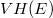$VH(E)$ and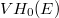$VH_0(E)$ of entire functions defined corresponding to a decreasing family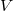$V$ of weights on a Banach space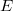$E$. For a suitably restricted family$V$ of weights, the bounded approximation property for a Banach space$E$ has been characterized in terms of the bounded approximation property for the space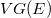$VG(E)$, the predual of the space$VH(E)$.

DOI Code: 10.1285/i15900932v37n1p53

Keywords: (LB)-spaces; weighted spaces of holomorphic functions; bounded approximation property; H\"{o}rmander algebras

Full Text: PDF